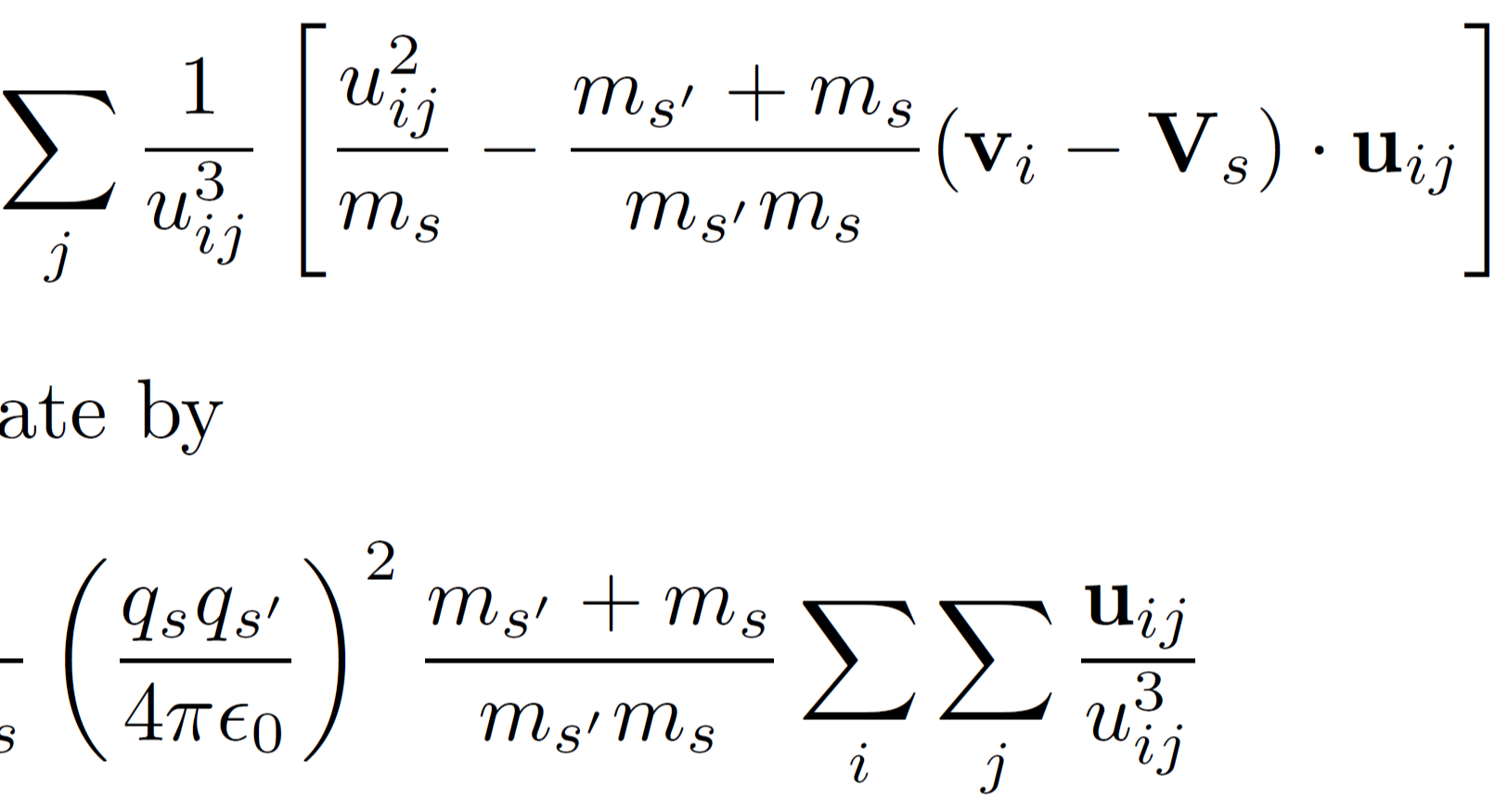# Efficient evaluation of collisional energy transfer terms for plasma particle simulations

plasma
high power lasers
simulations

Turrell, A. E., M. Sherlock, and S. J. Rose. “Efficient evaluation of collisional energy transfer terms for plasma particle simulations.” Journal of Plasma Physics 82, no. 1 (2016): 905820107. doi: `10.1017/S0022377816000131`Published

February 2016

## Abstract

Particle-based simulations, such as in particle-in-cell (PIC) codes, are widely used in plasma physics research. The analysis of particle energy transfers, as described by the second moment of the Boltzmann equation, is often necessary within these simulations. We present computationally efficient, analytically derived equations for evaluating collisional energy transfer terms from simulations using discrete particles. The equations are expressed as a sum over the properties of the discrete particles.

## Citation

``````@article{turrell2016efficient,
title={Efficient evaluation of collisional energy transfer terms for plasma particle simulations},
author={Turrell, AE and Sherlock, M and Rose, SJ},
journal={Journal of Plasma Physics},
volume={82},
number={1},
pages={905820107},
year={2016},
publisher={Cambridge University Press}
}``````